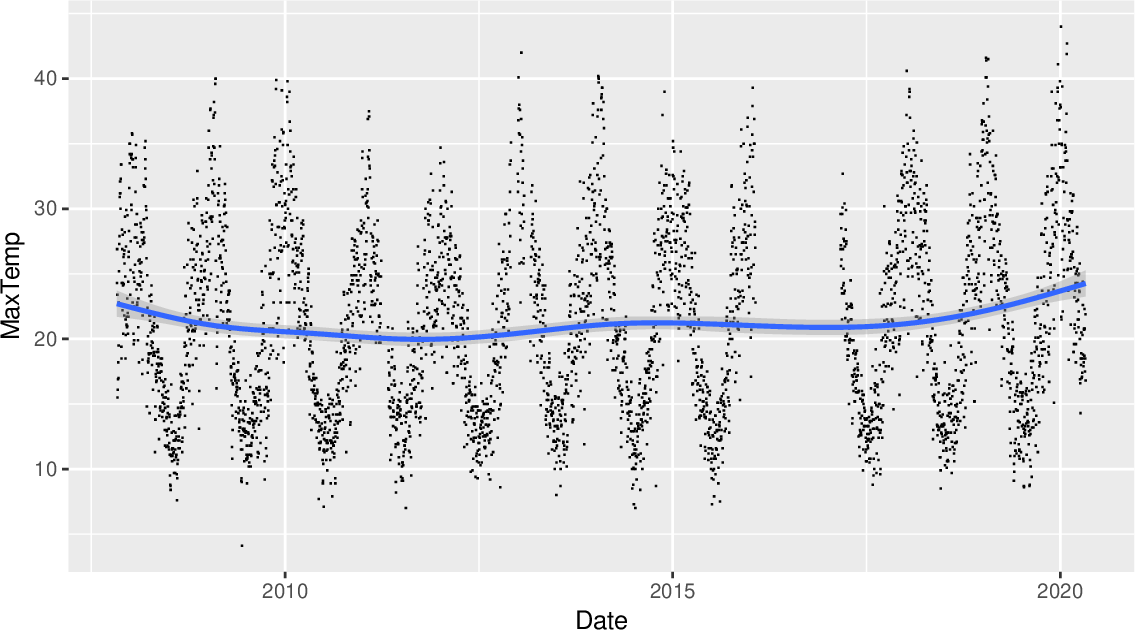Data Science Desktop Survival Guide by Graham WilliamsDesktop Survival Project Home Preface Data Science Introducing R R Constructs R Tasks R Strings R Read, Write, and Create Data Template Data Exploration Data Wrangling Data Visualisation Statistics ML Template ML Scenarios ML Activities ML Applications ML Algorithms Cluster Analysis Decision Trees Computer Vision Graph Data Privacy Literate Data Science Coding with Style Resources Bibliography Index

## Scatter Plot Smooth Gam

Rawds %>%   filter(location=="Canberra") %>%   ggplot(aes(x=date, y=max_temp)) +   geom_point(shape=".") +   geom_smooth(method="gam", formula=y~s(x, bs="cs")) +   labs(x=vnames["date"], y=vnames["max_temp"]) This scatter plot of x=date against y=max_temp shows a pattern of seasonality over the dataset and a trend line over the period of the dataset. The scatter plot is again created using ggplot2::geom_point(). Typical of scatter plots of big data there will be many overlaid points. To reduce the impact the points are reduced to a small dot using shape=`"."`. An additional layer of a smooth fitted curve, using ggplot2::geom_smooth(), is added. The dataset has many points and so a smoothing method recommended is method="gam" which will automatically be chosen if not specified but with a message to that effect. The formula specified using formula= is also the default for method="gam".